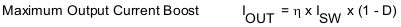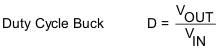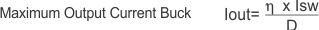SLVSB76B August   2012  – August 2019

PRODUCTION DATA.

1. Features
2. Applications
3. Description
1.     Device Images
4. Revision History
5. Pin Configuration and Functions
6. Specifications
7. Detailed Description
1. 7.1 Overview
2. 7.2 Functional Block Diagram
3. 7.3 Feature Description
4. 7.4 Device Functional Modes
8. Application and Implementation
1. 8.1 Application Information
2. 8.2 Typical Application
1. 8.2.1 Design Requirements
2. 8.2.2 Detailed Design Procedure
3. 8.2.3 Application Curves
9. Power Supply Recommendations
10. 10Layout
11. 11Device and Documentation Support
12. 12Mechanical, Packaging, and Orderable Information

• YFG|8

#### 8.2.2.4 Current Limit

To protect the device and the application, the average input current is limited internally on the IC. At nominal operating conditions, this current limit is constant. The current limit value can be found in the Electrical Characteristics table. The current limit varies depending on the input voltage. A curve of the input current varying with the input voltage is shown in Figure 5 and Figure 6 respectively showing the minimum and the maximum current limit expected depending on input and output voltage.

Given the average input current in Figure 5 is then possible to calculate the output current reached in boost mode using Equation 5 and Equation 6 and in buck mode using Equation 7 and Equation 8.

Equation 5.Equation 6.Equation 7.Equation 8.where

• η = Estimated converter efficiency (use the number from the efficiency curves or 0.80 as an assumption)
• f = Converter switching frequency (typical 2 MHz)
• L = Selected inductor value
• ISW = Minimum average input current (Figure 5)